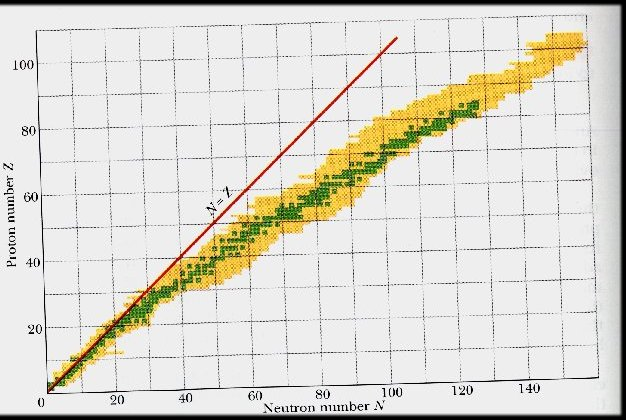# Nucleus stabilityThis article was checked by pedagogue, but more than year ago. Signature:  Carmeljcaruana (talk)
Article to be checked

Nucleus Stability

What is the nuclear stability? Nuclear stability means that nucleus is stable meaning that it does not spontaneously emit any kind of radiation. On the other hand, if the nucleus is unstable, it has the tendency of emitting some kind of radiation, which makes it radioactive. Therefore the radioactivity is associated with unstable nucleus:

We want to know why there is a radioactivity. What makes the nucleus stable? There are no concrete theories to explain this, but there are only general observations based on the available stable isotopes. It appears that neutron to proton ratio is the dominant factor in nuclear stability. Then how do we predict the nuclear stability? One of the simplest ways of predicting the nuclear stability is based on whether nucleus contains odd/even number of protons and neutrons:

Protons Neutrons Number of Stable Nuclides Stability
Odd Odd 4 least stable
Odd Example 50 less stable
Even Odd 57 more stable
Even Even 168 most stable

• Nuclides containing odd numbers of both protons and neutrons are the least stable means more radioactive.

• Nuclides containing even numbers of both protons and neutrons are most stable means less radioactive.

• Nuclides contain odd numbers of protons and even numbers of neutrons are less stable than nuclides containing even numbers of protons and odd numbers of neutrons.

The Valley of Nuclear StabilityNumber of protons and neutrons in nuclei

green square = stable nucleus